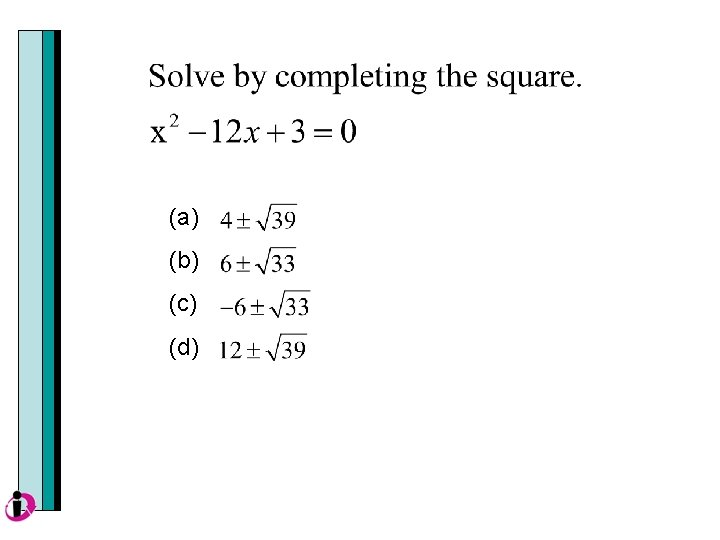• Slides: 35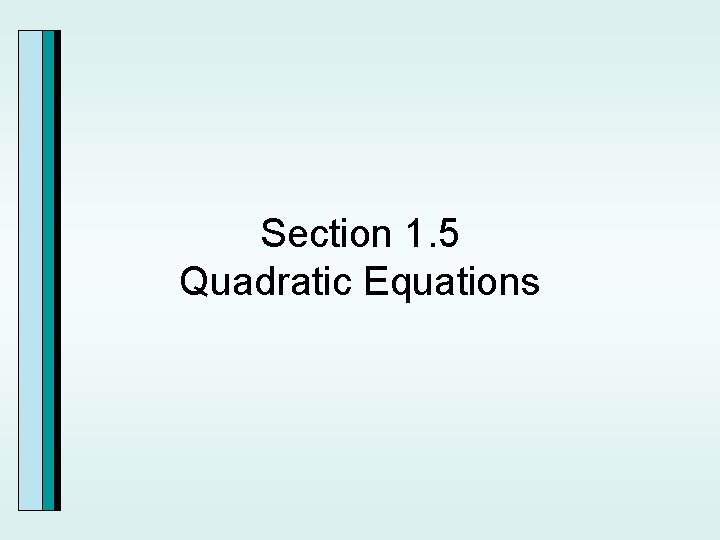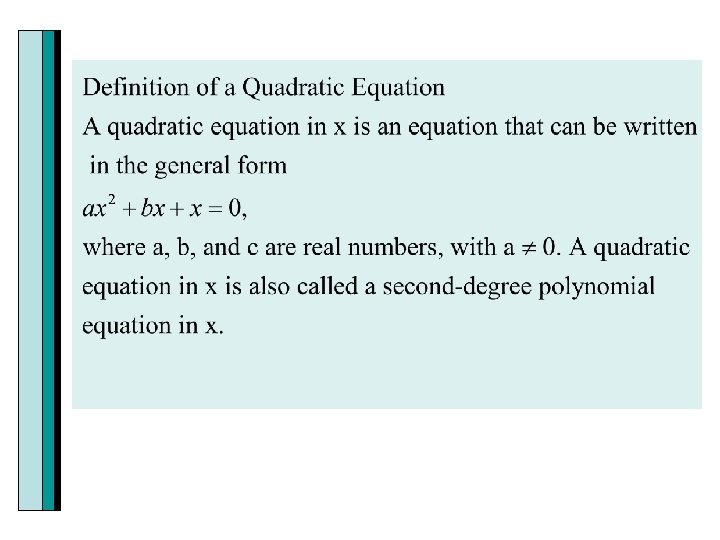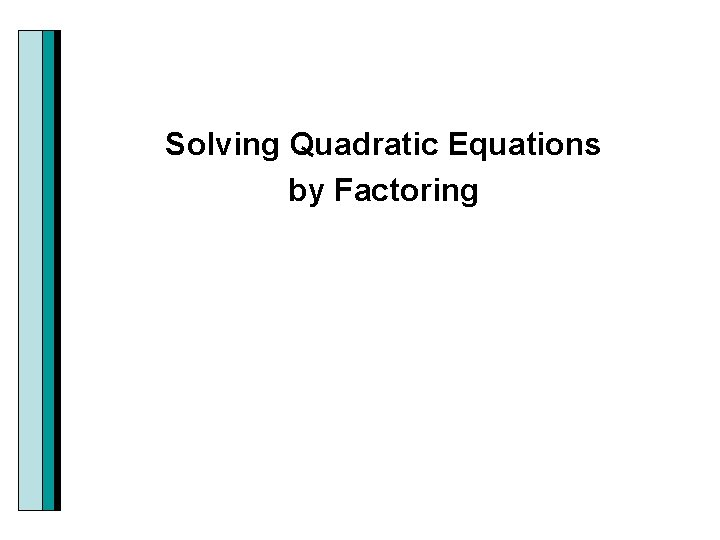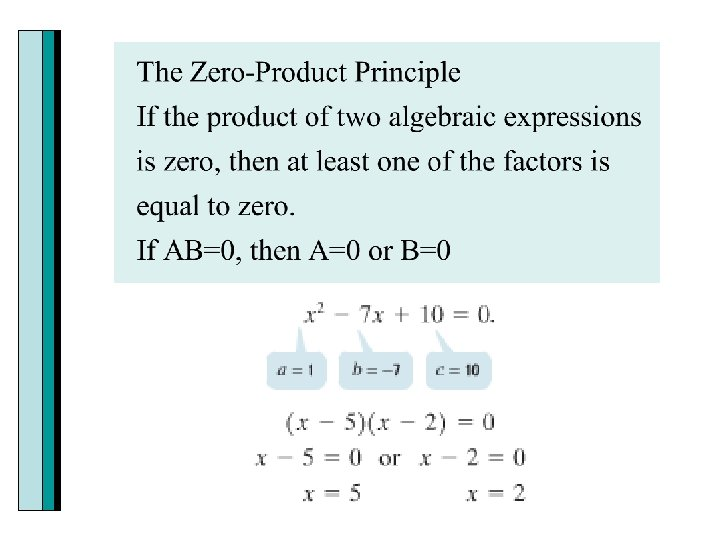Example Solve by factoring: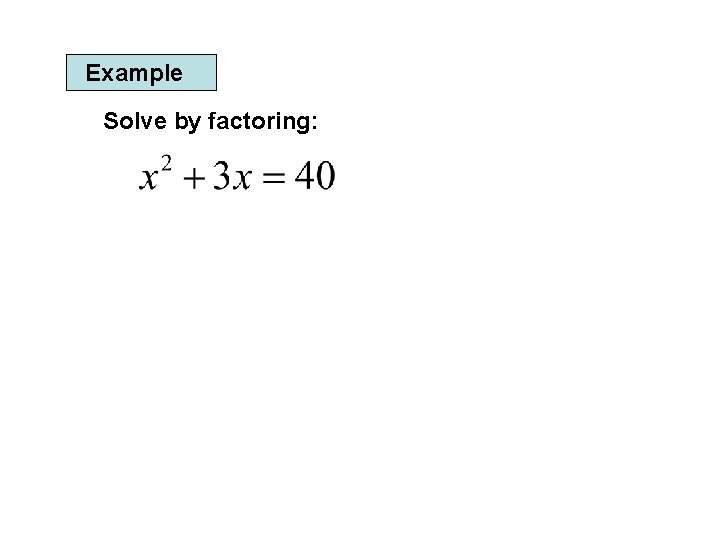Example Solve by factoring: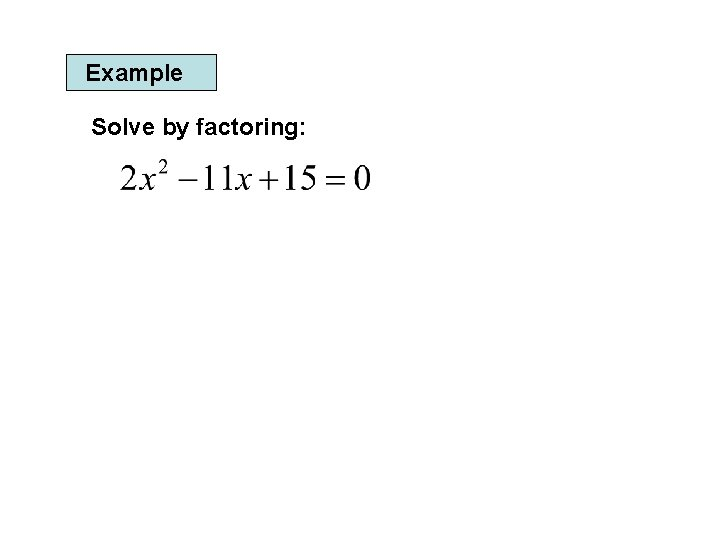Example Solve by factoring:Graphing Calculator The real solutions of a quadratic equation ax 2+bx+c=0 correspond to the x-intercepts of the graph. The U shaped graph shown below has two x intercepts. When y=0, the value(s) of x will be the solution to the equation. Since y=0 these are called the zeros of the function.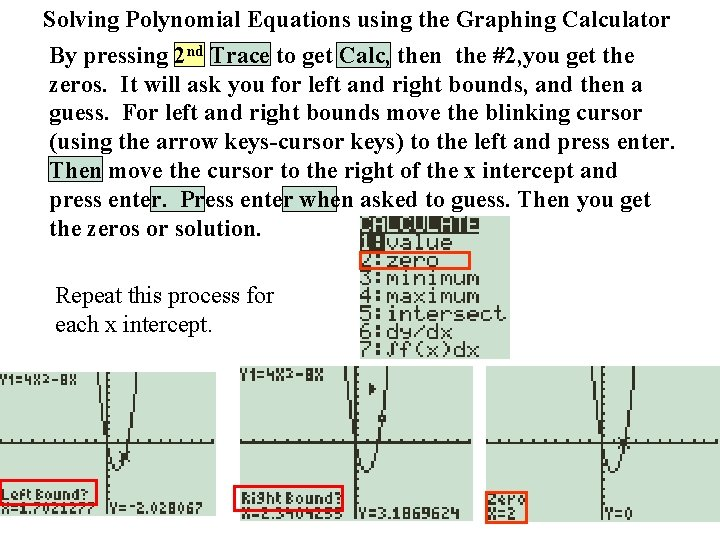Solving Polynomial Equations using the Graphing Calculator By pressing 2 nd Trace to get Calc, then the #2, you get the zeros. It will ask you for left and right bounds, and then a guess. For left and right bounds move the blinking cursor (using the arrow keys-cursor keys) to the left and press enter. Then move the cursor to the right of the x intercept and press enter. Press enter when asked to guess. Then you get the zeros or solution. Repeat this process for each x intercept.Solving Quadratic Equations by the Square Root PropertyExample Solve by the square root property: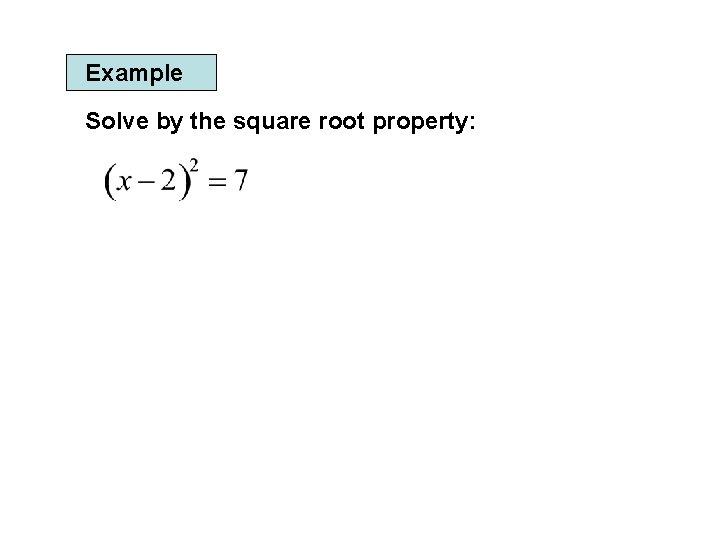Example Solve by the square root property: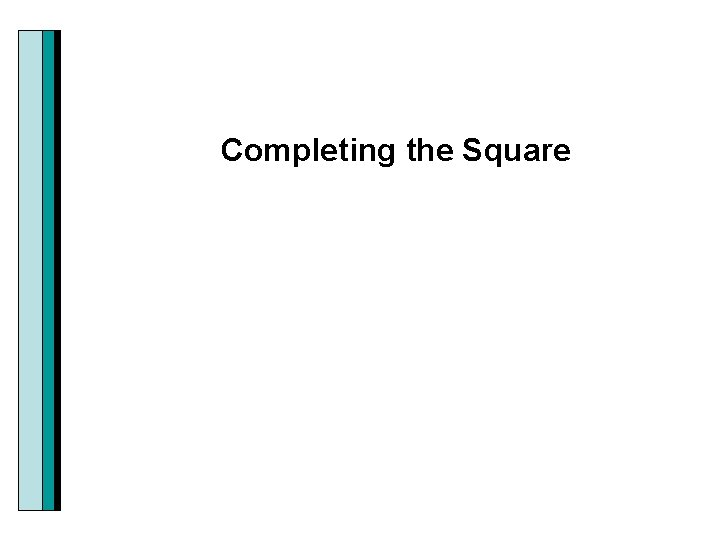Completing the Square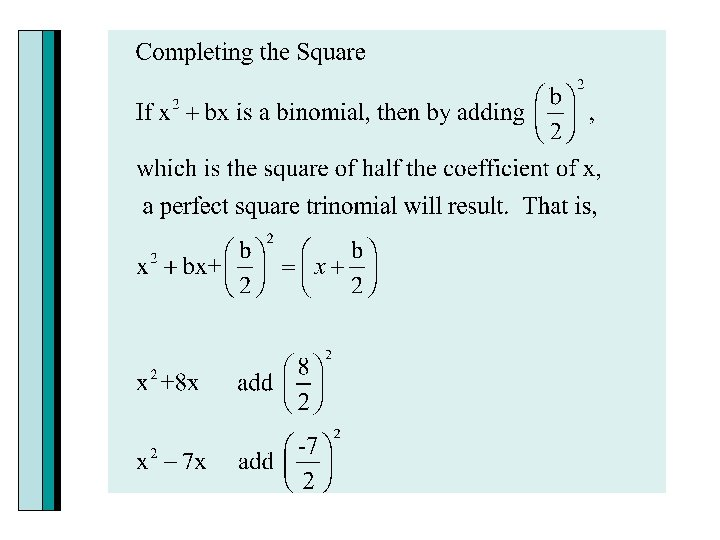Why we call this completing the square.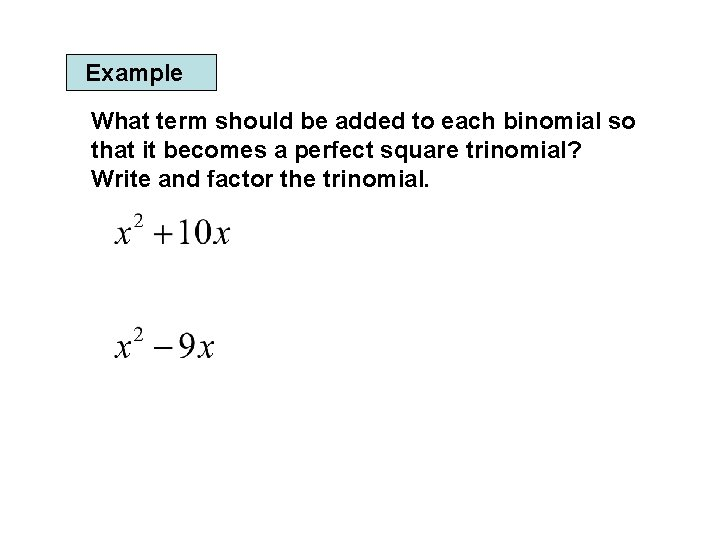Example What term should be added to each binomial so that it becomes a perfect square trinomial? Write and factor the trinomial.Example Solve by Completing the Square: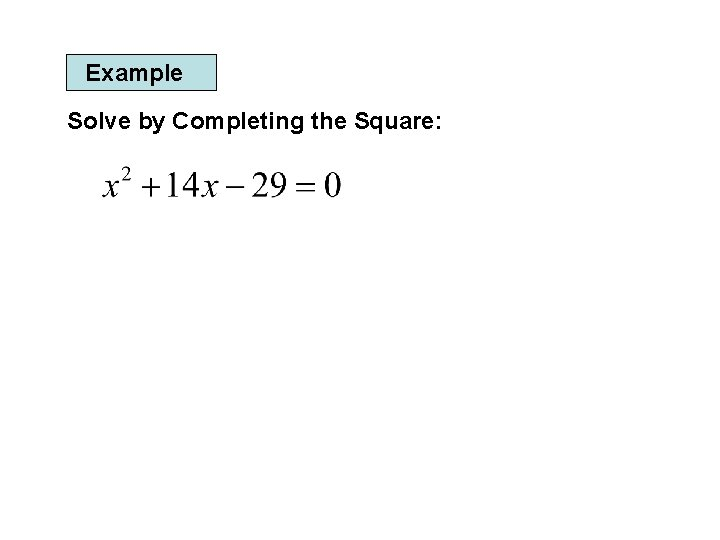Example Solve by Completing the Square: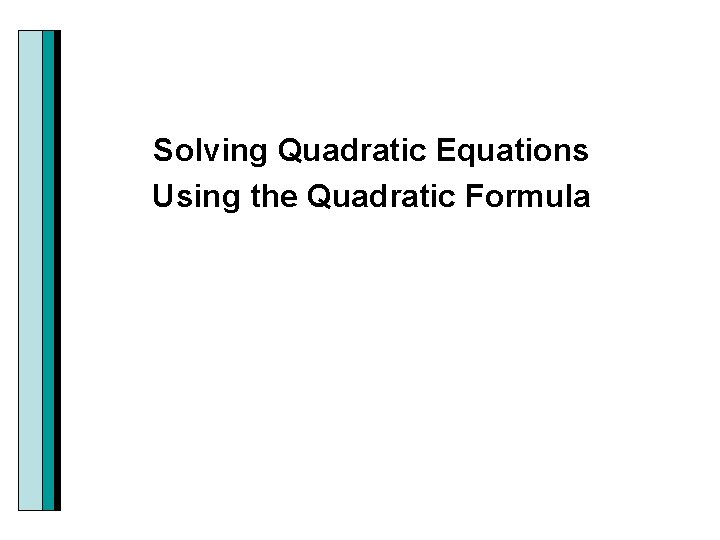Example Solve by using the Quadratic Formula: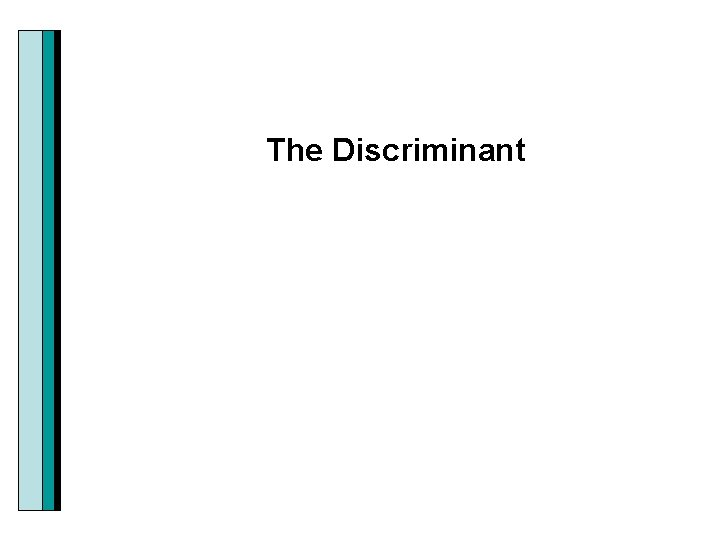The Discriminant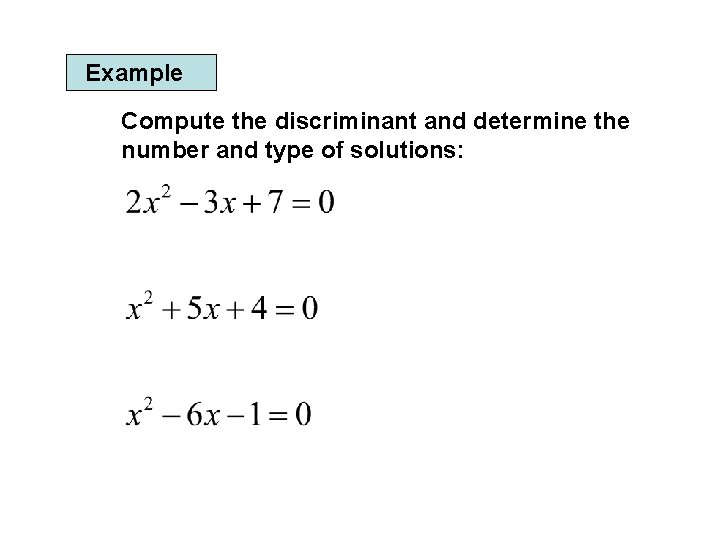Example Compute the discriminant and determine the number and type of solutions:Determining Which Method to Use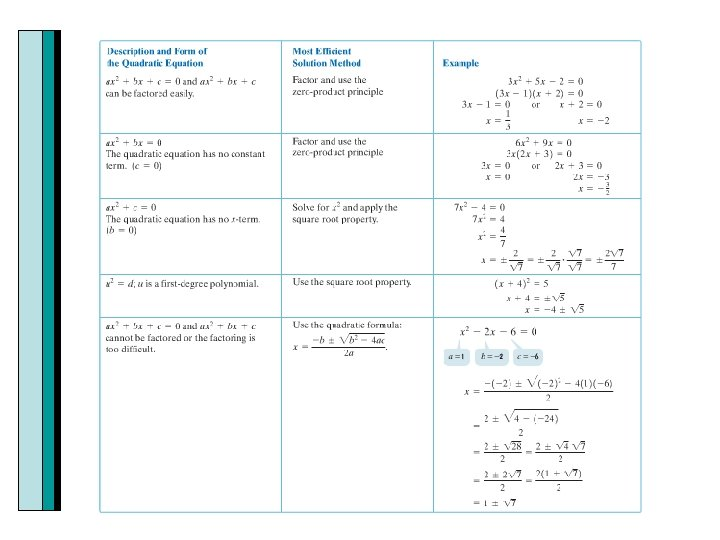Applications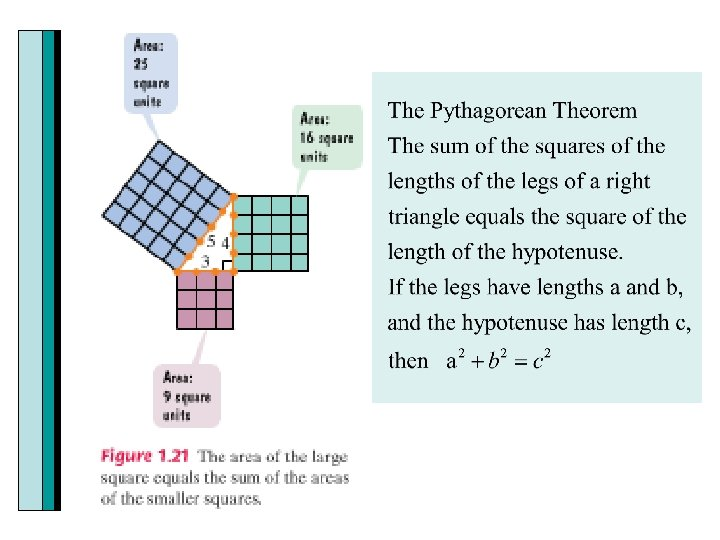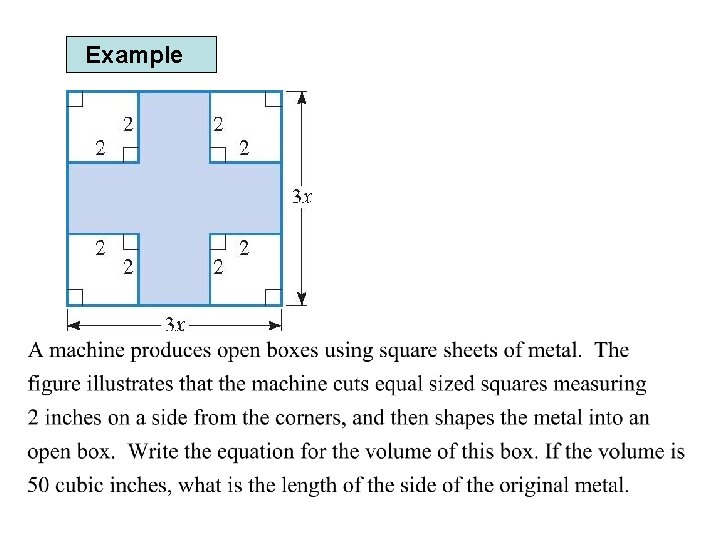Example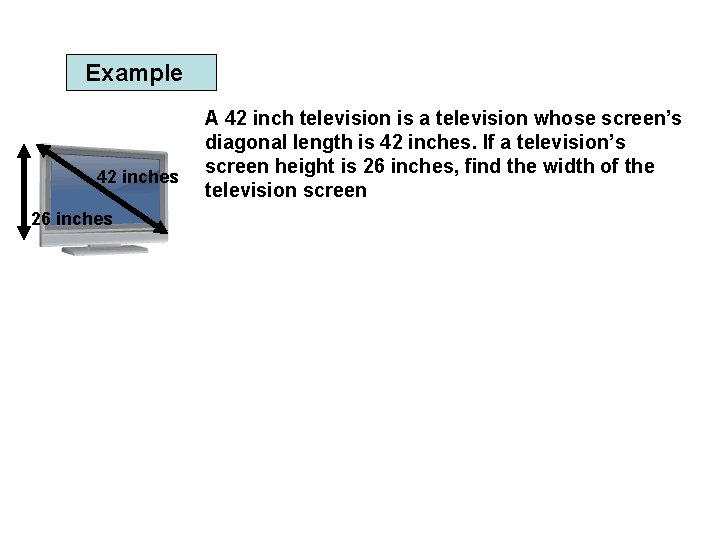Example 42 inches 26 inches A 42 inch television is a television whose screen’s diagonal length is 42 inches. If a television’s screen height is 26 inches, find the width of the television screen